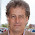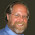## Wednesday, February 18, 2015

### How many samples do I need? (with lots of assumptions)

In an earlier post, I looked at the question "How many samples of a production run do I need to assure that 68% are within tolerance?" I concluded with "at least 300, and preferably 1,200. In the first pass I made only one assumption - that the sampling of parts to test was done at random. I answered the question with no information about the metric or about the process.

For my answer, I reduced the question down to a simple one. Suppose that a green M&M is put into a bucket/barrel/swimming pool whenever a good part is produced, and that a red M&M is put in whenever a bad part is produced. For the testing of this production run, M&Ms are pulled out at random, and noted as being either good or bad. After tallying the color of each M&M, they are replaced into the bucket/barrel/swimming pool.

Note the assumptions. I assume that the production run is sampled at run with replacement. And that's about it.

Statistics of color difference values

Today I answer the question with a whole lot of additional assumptions. Today I assume that the metric being measured and graded is the color difference value, in units of ΔE. And I make some assumptions about the statistical nature of color differences.

I assembled real-world data to create an archetypal cumulative probability density function (CPDF) of color difference data from a collection of 262 color difference data sets each with 300 to 1,600 data points. In total, my result is a distillation of  317,667 color differences from 201 different print devices, including web offset, coldset, flexo, and ink jet printers. So, a lot of data was reduced to a set of 101 percentile points shown in the image below. Note that this curve has been normalized to have a median value of 1.0 ΔE, on the assumption that all the curves have the same shape, but differ in scale.

Archetypal cumulative probability density function for color difference data (ΔEab)

For my analysis, it is assumed that all color difference data has this same shape. Note that if one has a data set of color difference data, it can be transformed to relate to this archetype by dividing all the color difference values by the median of the data set. In my analysis of the 262 data sets, this may not of been an excellent assumption, but then again, it was not a bad assumption.

The archetypal curve is based on data from printing test targets each with of hundreds of CMYK values, and not from production runs of 10,000 copies of a single CMYK patch. For this analysis, I make the assumption that run-time color differences behave kinda the same. I've seen data from a couple three press runs. I dunno, might not be such a good assumption.

Let's see... are there any other assumptions that I am making today? Oh yeah... I have based the archetypal CPDF on color difference data based on the original 1976 ΔE formula and not the 2000. Today, I don't know how much of a difference this makes. Some day, I might know.

Monte Carlo simulation of press runs

I did some Monte Carlo simulations with all the aforementioned assumptions. I was asking a variation on the question asked in the previous blog. Instead of asking what the how many samples were needed to make a reliable pass/fail call, I asked how many samples were needed to get a reliable estimate of the 68th percentile. Subtle difference, but that's the nature of statistics.

As in the previous blog, I will start with the example of the printer who pulls only three samples and from these three, determines the 68th percentile. I'm not sure just how you get a 68th percentile from only three samples, but somehow when I use the PERCENTILE function in Excel or the Quantile function in Mathematica, they give me a number. I assume that the number means something reasonable.

Now for a couple more assumptions. I will assume that the tolerance threshold is 4 ΔE (in other words, 68% must be less than 4 ΔE), and that the printer is doing a pretty decent job of holding this - 68% of the samples are below 3.5 ΔE. One would hope that the printer gets the thumbs up on the job almost all the time, right?

Gosh, that would be nice, but my Monte Carlo simulation says that this just ain't gonna happen. I ran the test 10,000 times. Each time, I drew three random samples from the archetypal CPDF shown above. From those, I calculated a 68th percentile. The histogram below shows the distribution of the 68th percentiles determined this way. Nearly 55% of the press runs were declared out of tolerance.

Distribution of estimates for the 68th percentile, determined from 3 random samples

There is something just a tad confusing here. The assumption was that the press runs had a 68th percentile of 3.5 ΔE. Wouldn't you expect that at least 50% of the runs were in tolerance? Yes, I think you might, but note two things: First, the distribution above is not symmetrical. Second, as I said before, determining the 68th percentile of a set of three data points is a bit of a slippery animal.

When this printer saw how many were failing, he asked for my advice. I pointed him to my previous blog, and he said "1200?!?!?  Are you kidding me!?!?  I can't even afford to measure 300 samples!" He ignored me, and never paid me my \$10,000 consulting fee, but I heard through the grapevine that he did start pulling 30 samples. That's why I get paid the big bucks. So people can ignore my advice.

Distribution of estimates for the 68th percentile, determined from 30 random samples

The image above shows what happened when he started measuring the color error on 30 samples per press run. Much better. Now only about 13% of the press runs are erroneously labelled "bad product". What happened after that depended on how sharp the teeth were in the contract between the printer and print buyer. Maybe the print buyer just shrugged it off when one out of every 8 print runs were declared out of tolerance? Maybe there's a lawsuit pending? I don't know. That particular printer never called me up with a status report.

What if the printer had heeded my advice and started pulling 300 samples to determine the 68th percentile? The results from one last Monte Carlo experiment are shown below. Here the printer pulled all 300 samples that I asked for. At the end of 10,000 press runs, the printer had only three examples where a good press run was called "bad".

Distribution of estimates for the 68th percentile, determined from 300 random samples

The previous examples were from the printer's perspective, where the printer responds with self-righteous indignation when sadistical control process has the gall to say that a good run is bad. We now turn this around and look at the print buyer's perspective.

Let's say that a printer is doing work that is not up to snuff... I dunno... let's say that the 68th percentile is at 4.5 ΔE. If the print buyer is a forgiving sort, then maybe this is OK by him. But then again, maybe his wife might tell him to stop being such a door mat?  (I am married to a woman who tells her spouse that all the time, especially when it comes to clients not paying.) We can't simulate what this print buyer's wife will tell him, but we can simulate how often statistical process control will erroneously tell him that a 4.5 ΔE run was good.

The results are similar, as I guess we would expect. If your vision of "statistical process control" means three samples, then 21.1% of the bad jobs will be given the rubber stamp of approval. The printer may like that, but I don't think the print buyer's spouse will stand for it.

If you up the sampling to 10 samples, quite paradoxically, the rate of mis-attribution goes up to 35.7%. That darn skewed distribution.

Pulling thirty samples doesn't help a great deal either. With 30 samples, the erroneous use of the "approved" stamp goes down only to 15.7%. If the count is increased to 100, then about 4.7% of the bad runs are called "good". But when 300 samples are pulled, the number drops way down to 0.06%.

Conclusions

I ran the simulation with a number of different sample sizes and a number of different underlying levels of "quality of production run".  The results are below. The percentages are the probability of making a wrong decision. In the first three lines of the table (3.0 ΔE to 3.75 ΔE), this is the chance that a good job will be called bad. In the next three lines of the table, this is the chance that a bad job will be called good.

 Actual 68th N = 3 N = 10 N = 30 N = 100 N = 300 3.0 ΔE 37.0% 4.0% 0.6% 0.0% 0.0% 3.5 ΔE 54.6% 18.1% 12.9% 1.5% 0.0% 3.75 ΔE 61.1% 29.0% 30.2% 13.2% 4.8% 4.25 ΔE 25.9% 47.5% 29.7% 20.9% 5.1% 4.5 ΔE 21.1% 35.7% 15.7% 4.7% 0.1% 5.0 ΔE 13.1% 19.6% 2.9% 0.0% 0.0%

Calculation of this table is a job for an applied math guy. Interpreting the table is a job for a statistician, which is at the edge of my competence. Deciding how to use this table is beyond my pay grade. It depends on how comfortable you are with the various outcomes. If, as a printer, you are confident that your process has a 68th percentile of 3.0 ΔE or less, then 30 samples should prove that point. And if your process slips a bit to the 3.5 ΔE level, and you are cool with having one out of eight of these jobs recalled, then don't let no one talk you into more than 30 samples. If you don't want those jobs recalled though...

If, as a print buyer, you really have no intention of cracking down on a printer until they hit the 5 ΔE mark, then you may be content with 30 samples. But if you want to have some teeth in the contract when a printer goes over 4.5 ΔE, then you need to demand at least 100 samples.

You will note that my answer was a little different than the previous blog post where I made minimal assumptions. If I make all the assumptions that are in this analysis, then the number of samples required (to demonstrate that 68% of the colors are within a threshold color difference) is smaller than the previous blog might  have suggested. Note that If one has a data set of color difference data, it can be transformed to relate to this archetype by dividing all the color difference values by the median of the data set. Then again, that one word ("assume", and its derivatives) in bold print has appears on this page 22 times...

In the first section, I mentioned "sampling with replacement", which means that you might sample a given product twice. Kind of a waste of time, really. Especially for small production runs, where the likelihood of duplicated effort is larger. Taken to the extreme, my conclusion was clearly absurd. Do I really need to pull 300 samples for my run of 50 units?!!?!?!

Well, no. Clearly one would sample a production run without replacement. But, in my world, a production run of 10,000 units is on the small side, so I admit to the myopic vision. For the purposes of this discussion, if the production run is over 10,000, it doesn't matter a whole lot whether a few of the 1,200 samples are measured twice.

1.2.